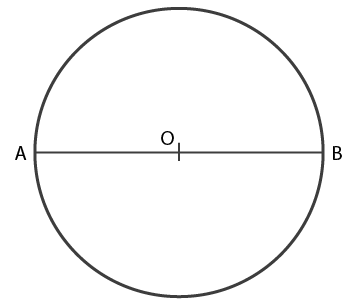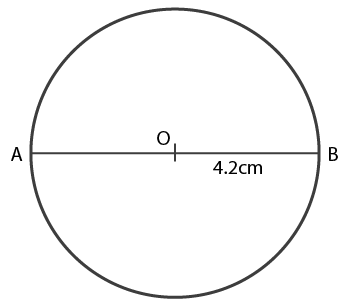# Draw a circle with diameter 6 cm. (i) 6 cm (ii) 8.4 cm. In each case measure the length of the radius of the circle drawn

(i) AB is the diameter of the circle

AB = 6 cm and

OA is the radius of the circleThe radius of the circle is,

(1/2) x 6 = 3 cm

Hence, OA = OB = 3 cm

(ii) AB is the diameter of the circle

AB = 8.4 cmOA is the radius of circle

(1/2) × 8.4 = 4.2 cm

Hence, OA = OB = 4.2 cm(0)(0)